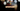# Leetcode | Solution of Isomorphic Strings in JavaScript

May 15th, 2020
|

In this post, we will solve Isomorphic Strings from leetcode and compute it's time and space complexities. Let's begin.

# Problem Statement

The question can be found at leetcode Isomorphic Strings problem.

The problem states that we are given two string and we need to determine if they are isomorphic.

# Solution

Isomorphic means that if we exchange each character of the alphabets by some other (unique) character, it should present the new string. For example, the word `add` and `egg` are isomorphic. Character `a` is replaced by `e` and character `d` is replaced by `g`. Word `ab` and `aa` are not isomorphic. Character `a` is replaced by character `a`, and the next character `b` is also replaced by character `a`. They have to be unique.

The approach is as follows

• We declare two maps to keep the pairs for string map from string 1 to 2 and string 2 to 1
• We will loop over string one and check if all the pairs in map one maps correctly to string two
• We will do the same with string 2
• If we find any mismatch anywhere, we return false as the string is not isomorphic
• If we reach the end of the program, it means strings were isomorphic. So we return true

We have discussed the approach, I urge you to go ahead on leetcode and give it another try.If you are here, it means something went wrong in implementation or you are just too lazy. In any case, let's see a simple implementation of the above logic.
``````var isIsomorphic = function (s, t) {
if (s.length !== t.length) return false;

const mapa = new Map();
const mapb = new Map();

for (let i = 0; i < s.length; i++) {
if (mapa.has(s[i])) {
if (mapa.get(s[i]) !== t[i]) {
return false;
}
} else {
mapa.set(s[i], t[i])
}

if (mapb.has(t[i])) {
if (mapb.get(t[i]) !== s[i]) {
return false;
}
} else {
mapb.set(t[i], s[i])
}
}

return true

};``````

Let's discuss the solution. It has two optimizations from our original discussion above

• If the strings are of different length they can't be isomorphic
• Since the first condition exists, we can merge both loops in one

So we took care of the condition in the second line. Next, we declared two maps and looped over a string(it can be any string since lengths are the same). In the body, we check if the mapping of the pairs is correct or not and we return accordingly.

It's a bit hard for me to explain the logic in writing here. I hope it makes sense, else please check the video below for more clarification.

Here are the stats on submission

``````Status: Accepted
Runtime: 120ms
Memory: 36.5MB``````

## Time and space complexity

### Time complexity

We are looping over the string array once, so time complexity would be O(n).

### Space complexity

We are using extra space for two maps. So space complexity is linear, O(m+n), where m and n are lengths of two strings

# Summary

So, we solved the Isomorphic Strings problem by using two pointer method and calculated the time and space complexities.

I hope you enjoyed solving this question. This is it for this one, complete source code for this post can be found on my Github Repo. Will see you in the next one.